Type Type system for a programming language set

• Slides: 14Type • Type system for a programming language = – set of types AND – rules that specify how a typed program is allowed to behave • Why? – to generate better code, with less runtime overhead – to avoid runtime errors – to improve expressiveness (see overloading) • How? – Type checking • Given an operation and an operand of some type, determine whether the operation is allowed on that operand – Type inference • Given the type of operands, determine – the meaning of the operation – the type of the operation • OR, without variable declarations, infer type from the way the variable is used 1Issues in typing • Does the language have a type system? – Untyped languages (e. g. assembly) have no type system at all • When is typing performed? – Static typing: At compile time – Dynamic typing: At runtime – Which is best? • How strictly are the rules enforced? – Strongly typed: No exceptions – Weakly typed: With well-defined exceptions • Type equivalence & subtyping – When are two types equivalent? • What does "equivalent" mean anyway? – When can one type replace another? 2Components of a type system • • Built-in types Rules for constructing new types Rules for determining if two types are equivalent Rules for inferring the types of expressions 3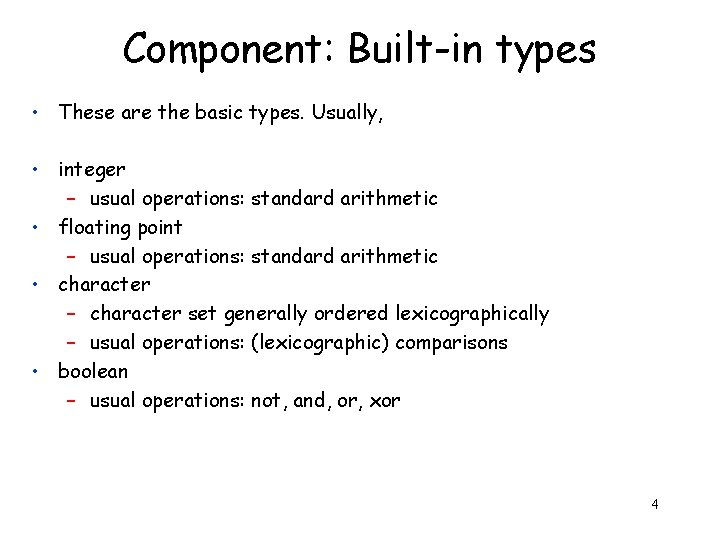Component: Built-in types • These are the basic types. Usually, • integer – usual operations: standard arithmetic • floating point – usual operations: standard arithmetic • character – character set generally ordered lexicographically – usual operations: (lexicographic) comparisons • boolean – usual operations: not, and, or, xor 4Component: type constructors • Arrays – array(I, T) denotes the type of an array with elements of type T, and index set I – multidimensional arrays are just arrays where T is also an array – operations: element access, array assignment, products • Strings – bitstrings, character strings – operations: concatenation, lexicographic comparison • Products – Groups of multiple objects of different types • essentially, Cartesian product of types (useful in functions later) • Records (structs) – Groups of multiple objects of different types where the elements are given specific names. 5Component: type constructors • Pointers – addresses – operations: arithmetic, dereferencing, referencing – issue: equivalency • Function types – A function such as "int add(real, int)" has type real int 6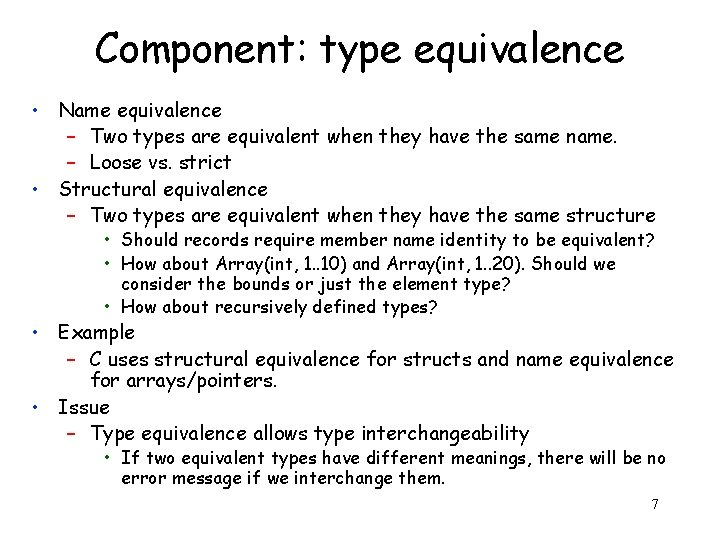Component: type equivalence • Name equivalence – Two types are equivalent when they have the same name. – Loose vs. strict • Structural equivalence – Two types are equivalent when they have the same structure • Should records require member name identity to be equivalent? • How about Array(int, 1. . 10) and Array(int, 1. . 20). Should we consider the bounds or just the element type? • How about recursively defined types? • Example – C uses structural equivalence for structs and name equivalence for arrays/pointers. • Issue – Type equivalence allows type interchangeability • If two equivalent types have different meanings, there will be no error message if we interchange them. 7Component: type equivalence • Subtyping – An operation that expects a type T and receives a type T' which is not equivalent to T, may still go ahead if T is a subtype of T'. – But what exactly is a subtype? • A subset of the values and superset of the properties? • Is a subclass a subtype? 8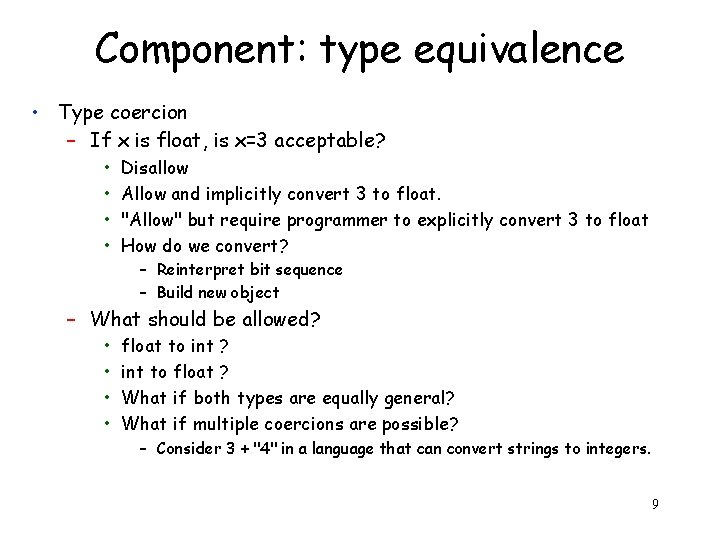Component: type equivalence • Type coercion – If x is float, is x=3 acceptable? • • Disallow Allow and implicitly convert 3 to float. "Allow" but require programmer to explicitly convert 3 to float How do we convert? – Reinterpret bit sequence – Build new object – What should be allowed? • • float to int ? int to float ? What if both types are equally general? What if multiple coercions are possible? – Consider 3 + "4" in a language that can convert strings to integers. 9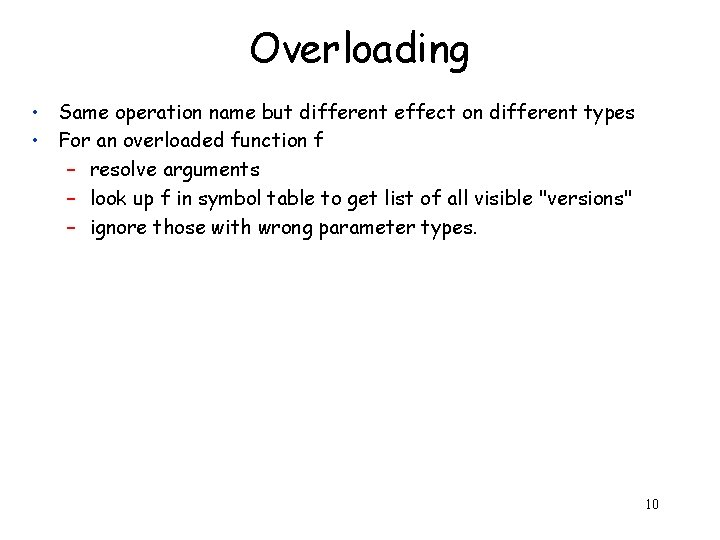Overloading • Same operation name but different effect on different types • For an overloaded function f – resolve arguments – look up f in symbol table to get list of all visible "versions" – ignore those with wrong parameter types. 10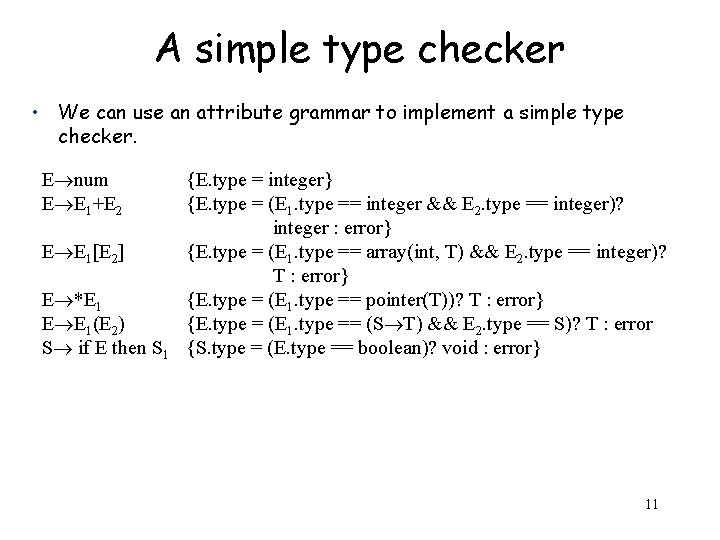A simple type checker • We can use an attribute grammar to implement a simple type checker. E num E E 1+E 2 E E 1[E 2] E *E 1 E E 1(E 2) S if E then S 1 {E. type = integer} {E. type = (E 1. type == integer && E 2. type == integer)? integer : error} {E. type = (E 1. type == array(int, T) && E 2. type == integer)? T : error} {E. type = (E 1. type == pointer(T))? T : error} {E. type = (E 1. type == (S T) && E 2. type == S)? T : error {S. type = (E. type == boolean)? void : error} 11Inference rules • The formal notation for type checking/inference is rules of inference • They have the form: Environment Hypotheses Environment Conclusions • If we can prove the hypotheses are all true in the existing environment, then the conclusion is also true. • For example: A E 1: int A A E 2: int E 1 + E 2 : int In English: If expressions E 1 and E 2 both have type int, then E 1+E 2 also has type int. 12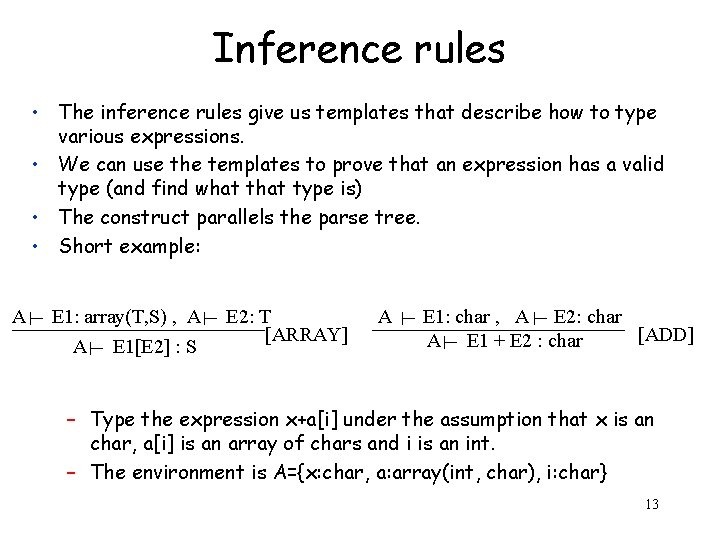Inference rules • The inference rules give us templates that describe how to type various expressions. • We can use the templates to prove that an expression has a valid type (and find what type is) • The construct parallels the parse tree. • Short example: A E 1: array(T, S) , A A E 1[E 2] : S E 2: T [ARRAY] A E 1: char , A E 2: char [ADD] A E 1 + E 2 : char – Type the expression x+a[i] under the assumption that x is an char, a[i] is an array of chars and i is an int. – The environment is A={x: char, a: array(int, char), i: char} 13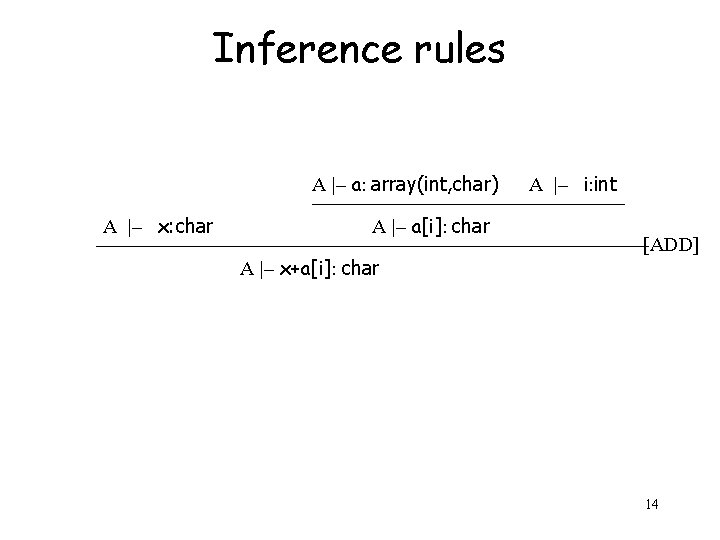Inference rules A |– a: array(int, char) A |– x: char A |– a[i]: char A |– x+a[i]: char A |– i: int [ADD] 14